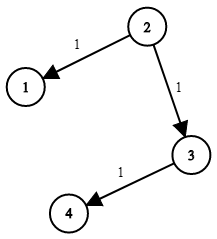# LeetCode Network Delay Time Solution

You are given a network of `n` nodes, labeled from `1` to `n`. You are also given `times`, a list of travel times as directed edges `times[i] = (ui, vi, wi)`, where `ui` is the source node, `vi` is the target node, and `wi` is the time it takes for a signal to travel from source to target.

We will send a signal from a given node `k`. Return the minimum time it takes for all the `n` nodes to receive the signal. If it is impossible for all the `n` nodes to receive the signal, return `-1`.

Example 1:Input: `times = [[2,1,1],[2,3,1],[3,4,1]], n = 4, k = 2`
Output: `2`

Example 2:
Input: `times = [[1,2,1]], n = 2, k = 1`
Output: `1`

Example 3:
Input: `times = [[1,2,1]], n = 2, k = 2`
Output: `-1`

Constraints:

• `1 <= k <= n <= 100`
• `1 <= times.length <= 6000`
• `times[i].length == 3`
• `1 <= ui, vi <= n`
• `ui != vi`
• `0 <= wi <= 100`
• All the pairs `(ui, vi)` are unique. (i.e., no multiple edges.)

## Solution

We wish to find the shortest path from `k` to all nodes in the graph. Dijkstra's algorithm is the best approach in finding shortest path from a single source. We will keep track of the delay time from `k` to each node, and find the maximum in the end.

#### Implementation

``````1def networkDelayTime(self, times: List[List[int]], n: int, k: int) -> int:
2    graph = defaultdict(list)
3    for src, dst, time in times:
4        graph[src].append((time, dst))
5
6    queue = [(0, k)]
7    delay_time = [inf for _ in range(n+1)]
8    while queue:
9        cur_time, cur_node = heapq.heappop(queue) # pop min from min-heap
10        if cur_time > delay_time[cur_node]:       # a shorter path exists
11            continue
12        for edge in graph[cur_node]:
13            new_time, new_node = edge
14            if delay_time[new_node] > cur_time + new_time:
15                delay_time[new_node] = cur_time + new_time
16                heapq.heappush(queue, (delay_time[new_node], new_node))
17
18    max_delay = max(delay_time)
19    return max_delay if max_delay != inf else -1``````